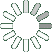Puzzle Command
Daily Puzzle
Number Logic #14
February 1, 2015Find a 6 digit number where:

The 1st digit is a multiple of 4.
The 2nd digit is a multiple of 2.
The 3rd digit is equal to 3.
The 4th digit is the sum of the 3rd and 5th digit.
The 5th digit is equal to 2.
The 6th digit is the sum of the 1st, 2nd, and 4th digit.

Puzzle Notes
6 digit numbers range from 100,000 to 999,999.
Enter in your solution below and click submit.